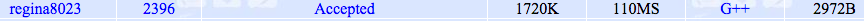# 【POJ 2396】 Budget

### Budget

Time Limit: 3000MS Memory Limit: 65536K
Total Submissions: 6317 Accepted: 2393 Special Judge
Description

We are supposed to make a budget proposal for this multi-site competition. The budget proposal is a matrix where the rows represent different kinds of expenses and the columns represent different sites. We had a meeting about this, some time ago where we discussed the sums over different kinds of expenses and sums over different sites. There was also some talk about special constraints: someone mentioned that Computer Center would need at least 2000K Rials for food and someone from Sharif Authorities argued they wouldn’t use more than 30000K Rials for T-shirts. Anyway, we are sure there was more; we will go and try to find some notes from that meeting.

And, by the way, no one really reads budget proposals anyway, so we’ll just have to make sure that it sums up properly and meets all constraints.
Input

The first line of the input contains an integer N, giving the number of test cases. The next line is empty, then, test cases follow: The first line of each test case contains two integers, m and n, giving the number of rows and columns (m <= 200, n <= 20). The second line contains m integers, giving the row sums of the matrix. The third line contains n integers, giving the column sums of the matrix. The fourth line contains an integer c (c < 1000) giving the number of constraints. The next c lines contain the constraints. There is an empty line after each test case.

Each constraint consists of two integers r and q, specifying some entry (or entries) in the matrix (the upper left corner is 1 1 and 0 is interpreted as “ALL”, i.e. 4 0 means all entries on the fourth row and 0 0 means the entire matrix), one element from the set {<, =, >} and one integer v, with the obvious interpretation. For instance, the constraint 1 2 > 5 means that the cell in the 1st row and 2nd column must have an entry strictly greater than 5, and the constraint 4 0 = 3 means that all elements in the fourth row should be equal to 3.
Output

For each case output a matrix of non-negative integers meeting the above constraints or the string “IMPOSSIBLE” if no legal solution exists. Put one empty line between matrices.
Sample Input

2

2 3
8 10
5 6 7
4
0 2 > 2
2 1 = 3
2 3 > 2
2 3 < 5

2 2
4 5
6 7
1
1 1 > 10
Sample Output

2 3 3
3 3 4

IMPOSSIBLE

Source

Tehran 2003 Preliminary

#include <iostream>
#include <algorithm>
#include <cstring>
#include <cstdio>
#include <cmath>
#include <cstdlib>
#include <queue>
#define inf 0x3f3f3f3f
#define M 500005
using namespace std;
int p;
int cnt,tot,h[M],du[M],d[M],cur[M],v[M],down,up;
int id,n,m,s,t,s1,t1;
struct edge
{
int from,to,cap,flow,ne;
}E[M];
void Clear()
{
for (int i=s;i<=t;i++)
h[i]=0,du[i]=0;
for (int i=1;i<=n;i++)
for (int j=1;j<=m;j++)
down[i][j]=0,up[i][j]=inf;
tot=1;
cnt=0;
}
{
E[++tot]=(edge){from,to,cap,0,h[from]};
h[from]=tot;
E[++tot]=(edge){to,from,0,0,h[to]};
h[to]=tot;
}
int Build()
{
p=tot-1;
for (int i=1;i<=n;i++)
for (int j=1;j<=m;j++)
if (down[i][j]>up[i][j]) return 0;
else
{
cnt+=down[i][j];
id[i][j]=tot-1;
}
return 1;
}
int bfs()
{
for (int i=0;i<=t;i++)
v[i]=0;
v[s]=1;
queue<int> q;
q.push(s);
d[s]=0;
while (!q.empty())
{
int x=q.front();
q.pop();
for (int i=h[x];i;i=E[i].ne)
{
edge e=E[i];
if (!v[e.to]&&e.cap>e.flow)
{
v[e.to]=1;
d[e.to]=d[x]+1;
q.push(e.to);
}
}
}
return v[t];
}
int dfs(int x,int a)
{
if (x==t||!a) return a;
int flow=0;
for (int &i=cur[x];i;i=E[i].ne)
{
edge &e=E[i];
if (d[e.to]!=d[x]+1) continue;
int f=dfs(e.to,min(a,e.cap-e.flow));
if (f>0)
{
e.flow+=f;
E[i^1].flow-=f;
a-=f;
flow+=f;
if (!a) break;
}
}
return flow;
}
int Dinic()
{
int flow=0;
while (bfs())
{
for (int i=s;i<=t;i++)
cur[i]=h[i];
flow+=dfs(s,inf);
}
return flow==cnt;
}
int main()
{
int T;
scanf("%d",&T);
while (T--)
{
scanf("%d%d",&n,&m);
s1=n+m+1,t1=n+m+2;
s=0,t=n+m+3;
Clear();
int sum1=0,sum2=0;
for (int i=1;i<=n;i++)
{
int x;
scanf("%d",&x);
sum1+=x;
}
for (int i=1;i<=m;i++)
{
int x;
scanf("%d",&x);
sum2+=x;
}
cnt=sum1+sum2;
int k;
scanf("%d",&k);
for (int i=1;i<=k;i++)
{
int x1,y1,j;
char ch;
scanf("%d%d%s%d",&x1,&y1,ch,&j);
int a,b,c,d;
a=b=x1,c=d=y1;
if (!a) a=1,b=n;
if (!c) c=1,d=m;
for (int x=a;x<=b;x++)
for (int y=c;y<=d;y++)
{
if (ch=='=')
down[x][y]=max(down[x][y],j),up[x][y]=min(up[x][y],j);
else if (ch=='>')
down[x][y]=max(down[x][y],j+1);
else up[x][y]=min(up[x][y],j-1);
}
}
if (sum1==sum2&&Build()&&Dinic())
{
for (int i=1;i<=n;i++)
{
printf("%d",down[i]+E[id[i]].flow);
for (int j=2;j<=m;j++)
printf(" %d",down[i][j]+E[id[i][j]].flow);
printf("\n");
}
}
else printf("IMPOSSIBLE\n");
printf("\n");
}
return 0;
}©️2019 CSDN 皮肤主题: 技术黑板 设计师: CSDN官方博客